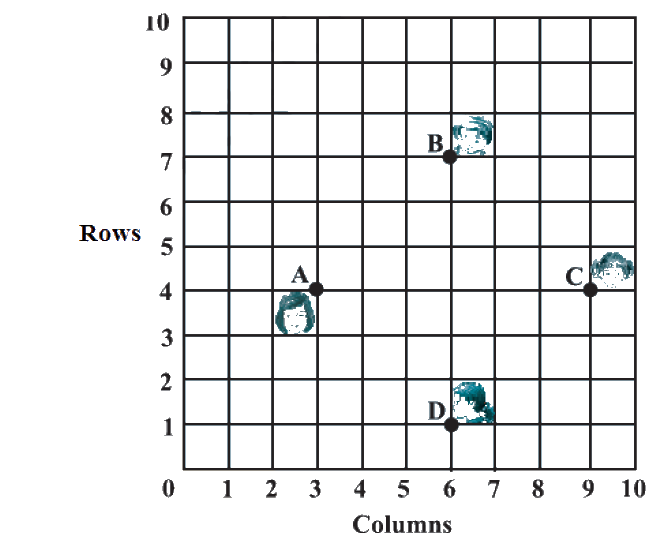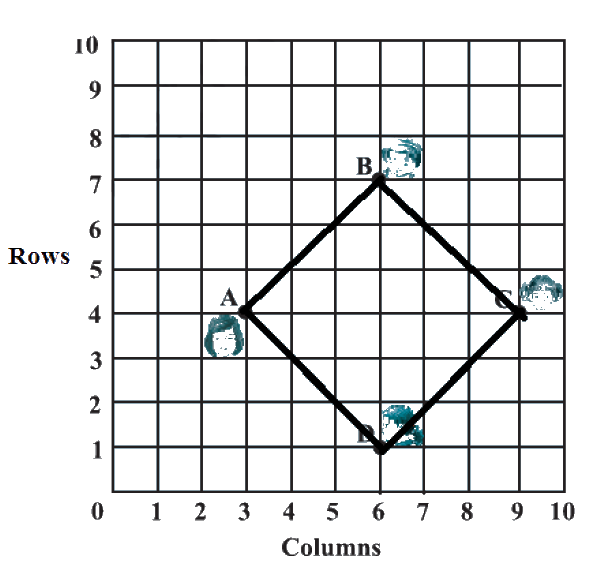# Ex.7.1 Q5 Coordinate Geometry Solution - NCERT Maths Class 10

## Question

In a classroom, $$4$$ friends are seated at the points $$A$$$$B$$, $$C$$ and $$D$$ as shown in the following figure. Champa and Chameli walk into the class and after observing for a few minutes Champa asks Chameli, “Don’t you think $$ABCD$$ is a square?” Chameli disagrees. Using distance formula, find which of them is correct.Video Solution
Coordinate Geometry
Ex 7.1 | Question 5

## Text Solution

Reasoning:

To prove that the points $$A$$,$$B$$,$$C$$ and $$D$$ from a square, the length of the four sides should be equal and the length of the two diagonals should be the same.

What is Known?

The $$x$$ and $$y$$ co-ordinates of the points between which the distance is to be measured can be deduced from the diagram.

What is Unknown?

To verify whether the positions of the four friends form a square or not.

Steps:

Let $$A \;(3, 4)$$, $$B\; (6, 7)$$, $$C \;(9, 4)$$, and $$D \;(6, 1)$$ be the positions of $$4$$ friends.

We know that the distance between the two points is given by the Distance Formula,

$\sqrt{\left(x_{1}-x_{2}\right)^{2}+\left(y_{1}-y_{2}\right)^{2}} \;\;\dots(1)$

To find $$AB$$ i.e. Distance between Points $$A \;(3, 4)$$ and $$B\; (6, 7)$$

• $$x_1 = 3$$
• $$y_1 = 4$$
• $$x_2 = 6$$
• $$y_2 =7$$

By substituting the values in the Equation (1), we get

\begin{align}AB &= \sqrt {{{(3 - 6)}^2} + {{(4 - 7)}^2}} \\ &= \sqrt {{{( - 3)}^2} + {{( - 3)}^2}} \\ &= \sqrt {9 + 9} \\ &= \sqrt {18} \\ &= 3\sqrt 2 \end{align}

To find $$BC$$ i.e. Distance between Points $$B (6, 7)$$ and $$C (9, 4)$$

• $$x_1 = 6$$
• $$y_1 = 7$$
• $$x_2 = 9$$
• $$y_2 = 4$$

By substituting the values in the Equation (1), we get

\begin{align}BC& = \sqrt {{{(6 - 9)}^2} + {{(7 - 4)}^2}} \\ &= \sqrt {{{( - 3)}^2} + {{(3)}^2}} \\ &= \sqrt {9 + 9} \\& = \sqrt {18} \\ &= 3\sqrt 2 \end{align}

To find $$CD$$ i.e. Distance between Points $$C \; (9, 4)$$ and $$D\; (6, 1)$$

• $$x_1 = 9$$
• $$y_1 = 4$$
• $$x_2 = 6$$
• $$y_2 = 1$$

By substituting the values in the Equation (1)

\begin{align}CB& = \sqrt {{{(9 - 6)}^2} + {{(4 - 1)}^2}} \\ &= \sqrt {{{(3)}^2} + {{(3)}^2}} \\ &= \sqrt {9 + 9} \\ &= \sqrt {18} \\ &= 3\sqrt 2 \end{align}

To find $$AD$$ i.e. Distance between Points $$B\; (3, 4)$$ and $$D \;(6, 1)$$

• $$x_1 = 3$$
• $$y_1 = 4$$
• $$x_2 = 6$$
• $$y_2 = 1$$

By substituting the values in the Equation (1)

\begin{align}AD &= \sqrt {{{(3 - 6)}^2} + {{(4 - 1)}^2}} \\ &= \sqrt {{{( - 3)}^2} + {{(3)}^2}} \\ &= \sqrt {9 + 9} \\&= \sqrt {18} \\ &= 3\sqrt 2 \end{align}

To find $$AC$$ i.e. Distance between Points $$A \;(3, 4)$$ and $$C\; (9, 4)$$

• $$x_1 = 3$$
• $$y_1 = 4$$
• $$x_2 = 9$$
• $$y_2 = 4$$

By substituting the values in the Equation (1), we get

\begin{align}{\text{Diagonal}}\,AC &\!=\! \sqrt {{{(3\! - \!9)}^2} \!+ \!{{(4\! - \!4)}^2}} \\ &= \sqrt {{{( - 6)}^2} + {0^2}} \\ &= 6\end{align}

To find $$BD$$ Distance between Points $$B \;(6, 7)$$ and $$D \;(6, 1)$$

• $$x_1 = 6$$
• $$y_1 = 7$$
• $$x_2 = 6$$
• $$y_2 = 1$$

By substituting the values in the Equation (1)

\begin{align}\text{Diagonal} \,BD &\!=\! \sqrt {{{\!(6 \!- \!6)}^2} \!+\! {{(7\! -\! 1)}^2}} \\ &= \sqrt {{0^2} + {{( - 6)}^2}} \\ &= 6\end{align}The four sides $$AB$$, $$BC$$, $$CD$$, and $$AD$$ are of same length and diagonals $$AC$$ and $$BD$$ are of equal length. Therefore, $$ABCD$$ is a square and hence, Champa was correct

Learn from the best math teachers and top your exams

• Live one on one classroom and doubt clearing
• Practice worksheets in and after class for conceptual clarity
• Personalized curriculum to keep up with school